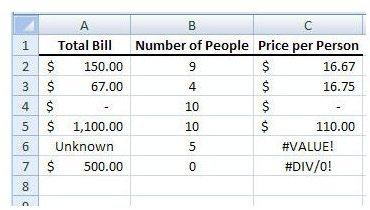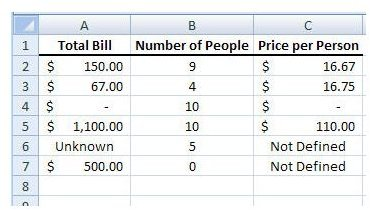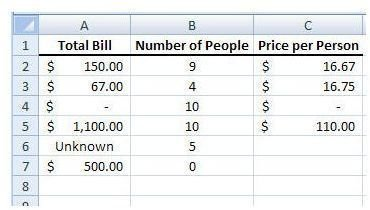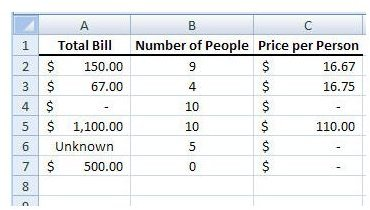# How to Use the IFERROR Function to Get Rid of DIV/0 Errors in Excel 2007

Page content

## #DIV/0 and #VALUE errors

One of the most efficient features available in Microsoft Excel is the ability to create a formula and then copy it into a large number of subsequent cells. For instance, if you have 500 rows of data and you want to add a new column that divides each number in column A by its associated value in column B, you only have to create that formula once. After creating the formula, you can copy and paste it into the remaining rows of the new column.

What if there are values in some of these rows that don’t make sense for the formula? Depending on the type of data, you’ll get either a #DIV/0 or a #VALUE error. One method to get rid of all these errors is to manually scan the spreadsheet and modify each cell that contains such an error. Another option that’s far more efficient is to use the IFERROR function when creating your original formula.

## The IFERROR Function

Despite its name, the IFERROR function is actually pretty simple in construction. This function is composed of two parts – the original function that you wanted to create (such as dividing two numbers) and a value to use when this function doesn’t make sense (like trying to divide by 0 or a text term like “bananas”). Probably the best way to illustrate this function is to use an example.

Let’s take a basic table of data that contains three columns. The total amount of a restaurant bill is in the first column, and the second column is comprised of the number of people in the party that received the bill. In the third column, we want to calculate the price per person. (Click the image below for a larger view.)

In normal circumstances, we could just construct a column that would divide the first column (column A in this example) by the second (column B). In other words, since our first row of data is actually in the second row of the worksheet, the formula for the first cell in the Price per Person column would be:

=A2/B2

Then, in a perfect world where all data entry is flawless, we could simply copy this formula and paste it into the remaining cells of that column. However, since our data does have some problems, we get the results shown in the following screenshot.Because the sixth row of the table contains text instead of a number we get the #VALUE error when trying to calculate our formula. Likewise, we get a #DIV/0 error in the last row of data, because the formula is trying to divide by 0.

Now, let’s see what happens if we modify the original formula and use the IFERROR function instead. Our function would look like this:

=IFERROR(A2/B2,”Not Defined”)

This function will return the value of the original function if it can be calculated. Otherwise, it will return the text value “Not Defined”.You can modify the second argument of the IFERROR function to be anything you wish. For instance, if you would prefer the cell to just be blank when the function cannot be evaluated, you can modify it to:

=IFERROR(A2/B2,””)

This would yield the results in the screenshot below.Tip: You need to include the quotation marks in the second argument of the IFERROR function if you want a text or “blank” value to be displayed. If you decide to use a numerical value instead, you can omit the quotation marks. For instance, if you want to display 0 whenever the function cannot be calculated, you could use the formula:

=IFERROR(A2/B2,0)

This result of this change in formula is shown below.## This post is part of the series: How to Use Logical Functions in Microsoft Excel 2007

The logical functions of Microsoft Excel 2007 are extremely powerful tools that be used for many applications. This group of articles takes a look at individual logical functions available in Excel and describes how they can be used to save you time and improve your project analysis.